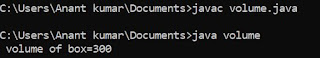## Wednesday, 4 July 2018

### C Function

When a program grow in size and complexity then we break that program into small module . These module are known as the function . Function increases the understandably of a program logic using function module independent logic can be defined .

Function provides the concept of modularity in a program which is best method to divide a complex program .

### Types of Function :

Function can be divided into two categories :

1. Library Function or  ( built in function )

2. User define or  ( Programmer define function )

1 . Library Function :

These function are the ready made function provides by the library of the compiler itself . some library function are as the follows .

A pow () ;-  This function is used to calculate the power of a given number this function works upon two arguments or parameter and after performing the operation always a integers types of variable . This required a header file for this function is < math.h>

Syntax :  int variable name = pow ( argument 1 , argument 2 ) ;

B . sqrt () ;-  This function is used to calculate the square root of a number . This function works on one argument and always return an integers type value .

Syntax :  int variable name = sqrt ( arg ) ;

C . toupper () ;  This function is used to convert a lower case character into upper case . the required header file is < c type.h >

Syntax :   character variable name = toupper ( char variable ) ;

D . tolower () ; This function is used to convert a upper case character into lower case required file    < c type .h >

syntax ; -  character variable name = tolower ( char variable ) ;

EXAMPLE :  Program power of two number using library function in C .

/* power of a number */
# include <stdio.h>
# include <math.h>
void main ()
{
int a ,b , c ;
clrscr () ;
printf ("\n Enter value of a:") ;
scanf ("%d", & a) ;
printf ("\n Enter value of b:") ;
scanf ("%d", & b) ;
c = pow ( a , b ) ;
printf ("\n %d", c) ;
getch () ;
}

EXAMPLE :  Program to convert a upper case character into lower case character  in C .

# include <stdio.h>
# include <math.h>
void main () ;
{
char ch ;
clrscr () ;
printf ("\n Enter a upper case character:") ;
scanf ("%d", & ch) ;
printf ("%c", tolower ( ch) ) ;
getch () ;
}

User define function in next page